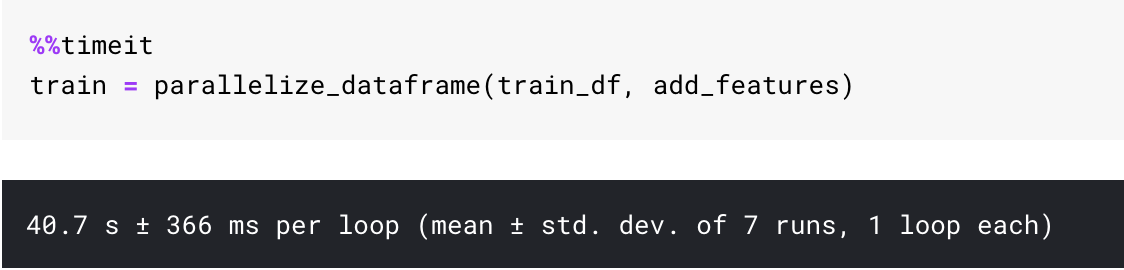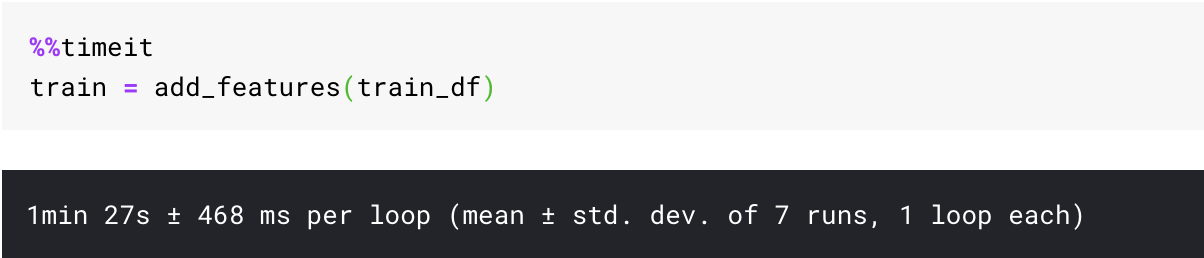Programming Data Science

# Make your own Super Pandas using MultiprocParallelization is awesome.

We data scientists have got laptops with quad-core, octa-core, turbo-boost. We work with servers with even more cores and computing power.

But do we really utilize the raw power we have at hand?

Instead, we wait for time taking processes to finish. Sometimes for hours, when urgent deliverables are at hand.

Can we do better? Can we get better?

In this series of posts named Python Shorts , I will explain some simple constructs provided by Python, some essential tips and some use cases I come up with regularly in my Data Science work.

This post is about using the computing power we have at hand and applying it to the data structure we use most.

## Problem Statement

We have got a huge pandas data frame, and we want to apply a complex function to it which takes a lot of time.

For this post, I will use data from the Quora Insincere Question Classification on Kaggle, and we need to create some numerical features like length, the number of punctuations, etc. on it.

The competition was a Kernel-based competition and the code needed to run in 2 hours. So every minute was essential, and there was too much time going in preprocessing.

Can we use parallelization to get extra performance out of our code?

Yes, we can.

## Parallelization using just a single functionCan we make all our cores run?

Let me first start with defining the function I want to use to create our features. add_features is the toy function we wish to apply to our data.

import random
import pandas as pd
import numpy as np
from multiprocessing import  Pool

df['question_text'] = df['question_text'].apply(lambda x:str(x))
df["lower_question_text"] = df["question_text"].apply(lambda x: x.lower())
df['total_length'] = df['question_text'].apply(len)
df['capitals'] = df['question_text'].apply(lambda comment: sum(1 for c in comment if c.isupper()))
df['caps_vs_length'] = df.apply(lambda row: float(row['capitals'])/float(row['total_length']),
axis=1)
df['num_words'] = df.question_text.str.count('\S+')
df['num_unique_words'] = df['question_text'].apply(lambda comment: len(set(w for w in comment.split())))
df['words_vs_unique'] = df['num_unique_words'] / df['num_words']
df['num_exclamation_marks'] = df['question_text'].apply(lambda comment: comment.count('!'))
df['num_question_marks'] = df['question_text'].apply(lambda comment: comment.count('?'))
df['num_punctuation'] = df['question_text'].apply(lambda comment: sum(comment.count(w) for w in '.,;:'))
df['num_symbols'] = df['question_text'].apply(lambda comment: sum(comment.count(w) for w in '*&\$%'))
df['num_smilies'] = df['question_text'].apply(lambda comment: sum(comment.count(w) for w in (':-)', ':)', ';-)', ';)')))
df['num_sad'] = df['question_text'].apply(lambda comment: sum(comment.count(w) for w in (':-<', ':()', ';-()', ';(')))
df["mean_word_len"] = df["question_text"].apply(lambda x: np.mean([len(w) for w in str(x).split()]))
return df


We can use parallelized apply using the below function.

def parallelize_dataframe(df, func, n_cores=4):
df_split = np.array_split(df, n_cores)
pool = Pool(n_cores)
df = pd.concat(pool.map(func, df_split))
pool.close()
pool.join()
return df


What does it do? It breaks the dataframe into n_cores parts, and spawns n_cores processes which apply the function to all the pieces.

Once it applies the function to all the split dataframes, it just concatenates the split dataframe and returns the full dataframe to us.

### How can we use it?

It is pretty simple to use.

train = parallelize_dataframe(train_df, add_features)


### Does this work?

To check the performance of this parallelize function, I ran %%timeit magic on this function in my Jupyter notebook in a Kaggle Kernel.vs. just using the function as it is:As you can see I gained some performance just by using the parallelize function. And it was using a kaggle kernel which has only got 2 CPUs.

In the actual competition, there was a lot of computation involved, and the add_features function I was using was much more involved. And this parallelize function helped me immensely to reduce processing time and get a Silver medal .

Here is the kernel with the full code.

## Conclusion

Parallelization is not a silver bullet; it is buckshot. It won’t solve all your problems, and you would still have to work on optimizing your functions, but it is a great tool to have in your arsenal.

Time never comes back, and sometimes we have a shortage of it. At these times we should be able to use parallelization easily.

# Parallelization is not a silver bullet it is a buckshot

Also if you want to learn more about Python 3, I would like to call out an excellent course on Learn Intermediate level Python from the University of Michigan. Do check it out.

I am going to be writing more beginner friendly posts in the future too. Let me know what you think about the series. Follow me up at <strong>Medium</strong> or Subscribe to my <strong>blog</strong> to be informed about them. As always, I welcome feedback and constructive criticism and can be reached on Twitter @mlwhiz .